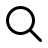# Knowledge Fusion- A Preparation Guide to Achieve|IBPS SBI RRB SSC exams

Daily Preparation Material & Quizzes for General Awareness,Computer,Banking,GS and many more...## The following pic represents solving possibility syllogisms without Venn diagrams. we will come to it shortly.

There are 14 basic formulas you need to learn in order to solve syllogisms without Venn diagrams.
The following must be learned.Here we go .

Examples of syllogisms solved without using venn diagram ( solved using formula based).

• All A are B  = Some A are B = Some B are A
• All A are B != All B are A
• All A are B != Some A are not B != Some B are Not A
• All A are B != No A are B != No B are A

• Some A are B  = Some B are A
• Some A are B != All B are A != All A are B
• Some A are B != No B are A != No A are B
• Some A are B != Some B are not A

• No A are B  = No B are A
• No A are B != Some B are Not A {Very important syllogisms shortcut}
• No A are B != Some A are Not B {Very important syllogisms shortcut}
• No A are B != All A are B

• Some A are not B  = No B are A {Very important syllogisms shortcut}
• Some A are not B != No A are B  {{Very important syllogisms shortcut}

Lets get into the learning method of syllogism shortcut..

Solved Example :-

• All  A are  B   {Take "All" From this}
• No  B are C   {Take "No" from this }
Add both we get "All + No"  for this  " All+No = No "
• Ans:- No A is C
Here ' No ' means we can derive a conclusion between A and C as "No A is C"
Observe that we have eliminated  B  which is common to both.